# Unsupervised learning can learn from features without label

Last updated on：7 months ago

Unsupervised learning can learn features by specifically designed loss without any pre-labels. There are two most common seen unsupervised learning methods, which are autoencoder and GANs. In this article, we mainly focus on autoencoder.

# Introduction

Unsupervised learning is about problems where we don’t have labelled answers, such as clustering, dimensionality reduction, and anomaly detection.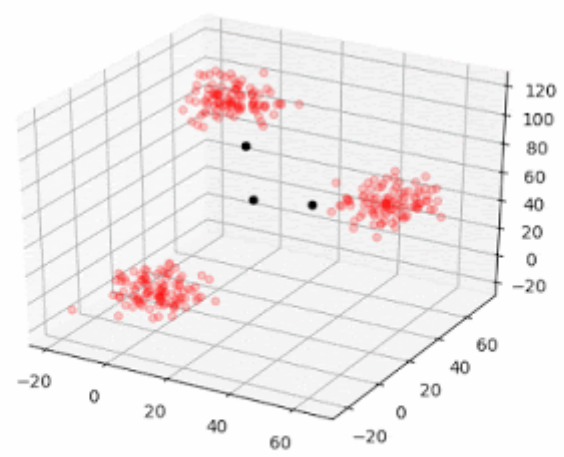Unsupervised learning is usually employed (e.g., clustering) to separate the images into two sets or clusters based on some inherent features of the pictures like colour, size, shape, etc.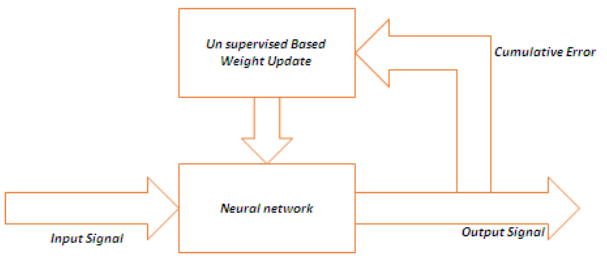## Motivation

• It is not easy to obtain a large amount of labelled data in practice, but it is easy to get a large amount of unlabelled data.
• Learning a suitable feature extractor using unlabelled data and then learning the classifier using labelled data can improve the performance.

## From the data point of view

Data in both input $x$ and output $y$ (learn the mapping $f$)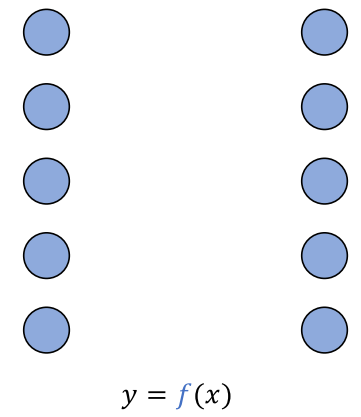# Autoencoder

Autoencoder would be an unsupervised learning method if we considered the features the “output”. Autoencoder is also a self-taught learning method, a type of artificial neural network used to learn efficient coding of unlabelled data. The encoding is validated and refined by attempting to regenerate the input from the encoding.

Word2Vec is another unsupervised, self-taught learning example.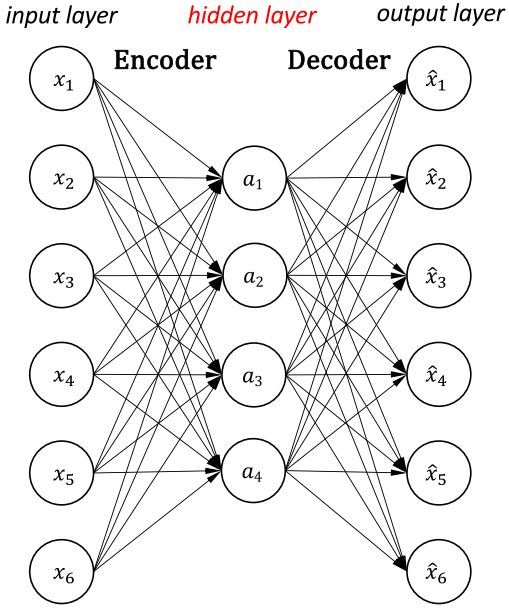Given M data samples,

$$\mathcal{L}_{\text{MSE}} = \frac{1}{M} \sum _{m=1}^M ||\hat{x}^m - x^m|| ^2_2$$

It is trying to learn an approximation to the identity function so that the input is “compress“ to the “compressed” features, discovering interesting structure about the data.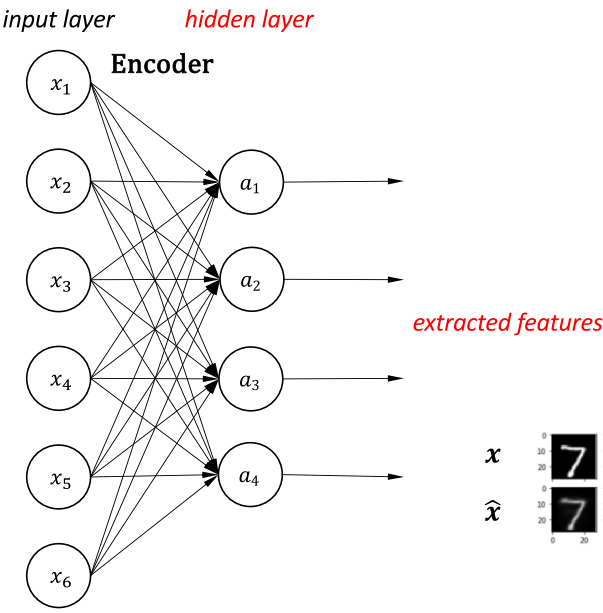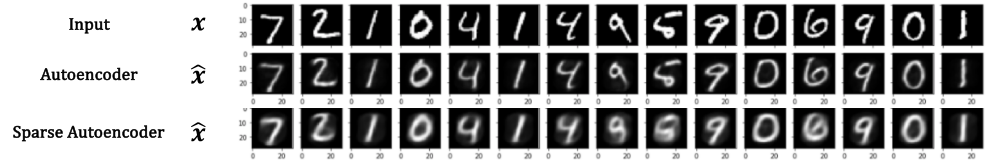## Sparse autoencoder

Even when the number of hidden units is large (perhaps even more significant than the number of input pixels), we can still discover an interesting structure, by imposing other constraints on the network.

In particular, if we impose a “sparsity” constraint on the hidden units, then the autoencoder will still discover exciting structures in the data, even if the number of hidden units is significant.

One neuron = one feature extractor.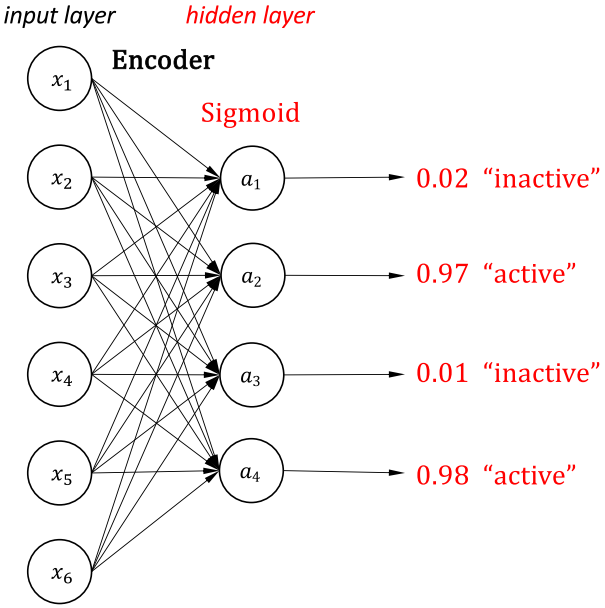Given M data samples and Sigmoid activation function, the active ratio of a neuron $a_j$:

$$\hat{\rho} _j = \frac{1}{M}\sum _{m=1}^{M}a_j$$

This makes the output “sparse”. We would like to enforce the following constraint, where $\rho$ is a “sparsity parameter“, such as 0.2 (20% of the neurons)

$$\hat{\rho}_j = \rho$$

The penalty term is as follow, where $s$ is the number of output neurons.

$$\mathcal{L}=\sum^S _{j=1}KL(\rho||\hat{\rho} _j)=\sum^S _{j=1} (\rho\text{log} \frac{\rho}{\hat{\rho} _j} + (1-\rho)\text{log}\frac{1-\rho}{1-\hat{\rho} _j}$$

The total loss:

$$\mathcal{L} _{\text{total}} = \mathcal{L} _{\text{MSE}} + \mathcal{L} _{\rho}$$

Method Hidden activation Reconstruction activation Loss function
method1 Sigmoid Sigmoid $$\mathcal{L} _{\text{total}} = \mathcal{L} _{\text{MSE}} + \mathcal{L} _{\rho}$$
method2 ReLU Softplus $$\mathcal{L}_{\text{total}} = \mathcal{L} _{\text{MSE}} + ||\bf{a}||$$

$||\bf{a}||$ is $\mathcal{L}_1$ on the hidden activation output.

## Other types

Denoising autoencoder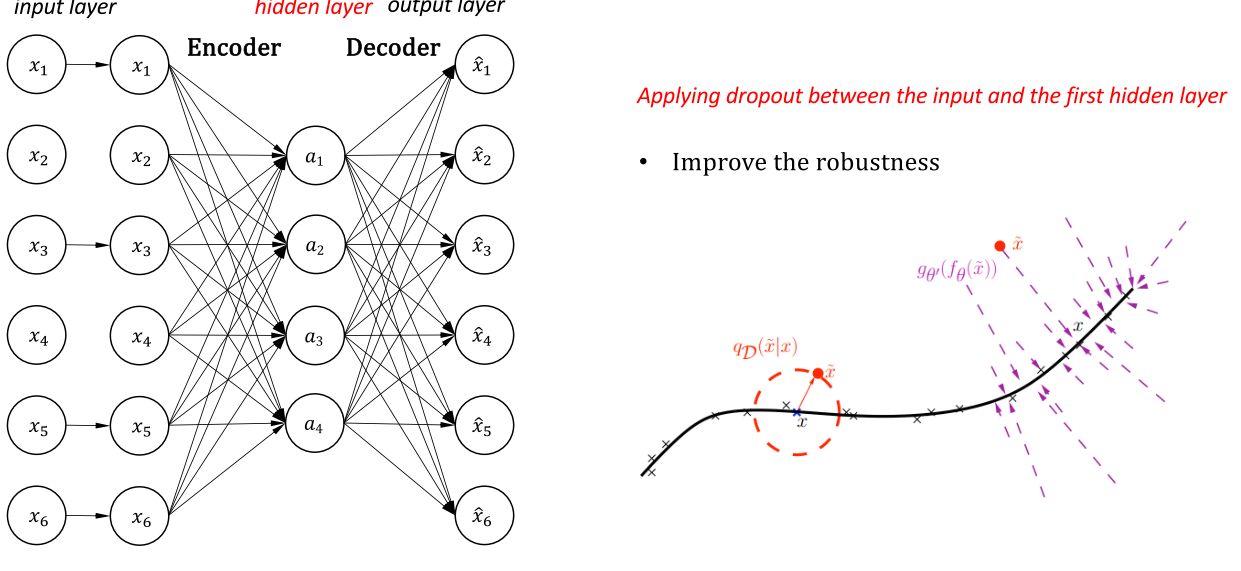Stacked autoencoder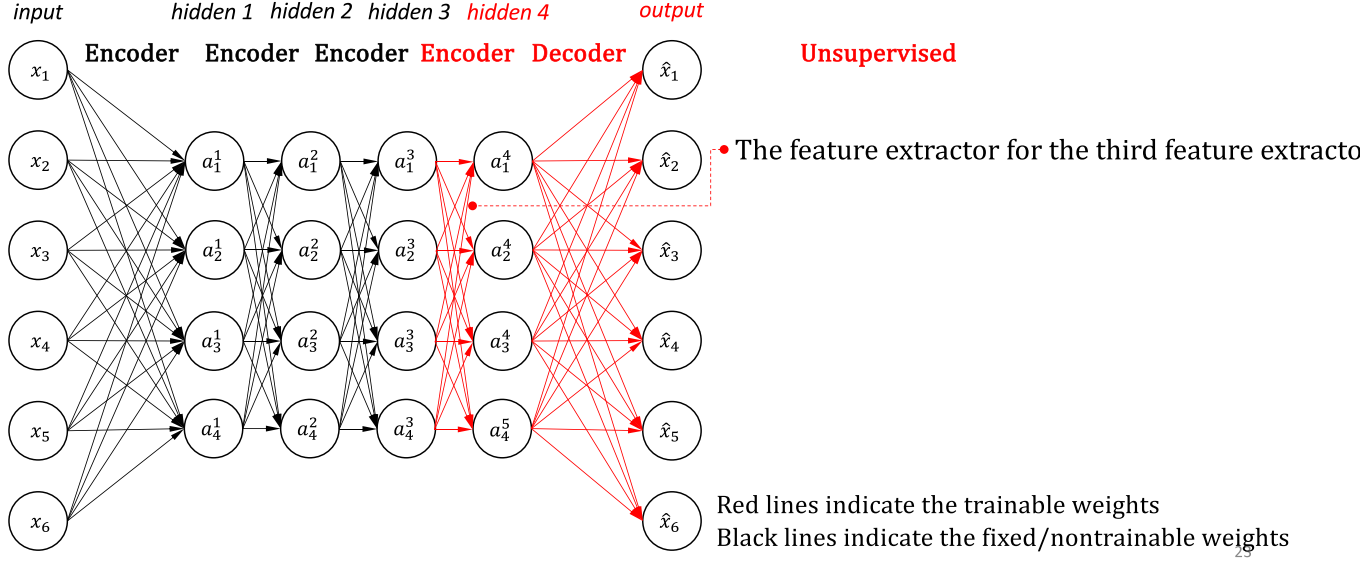Variational autoencoder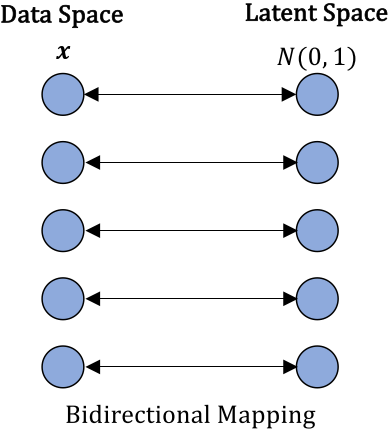# Applications

## Learn segmentation via domain adaptation

Each encoder receives a different augmented version of the input image, generated by $f_T$. The loss:$$\mathcal{L}_{\text{SRMA}} = \mathcal{L} _{\text{IND}} + \mathcal{L} _{\text{CRD}}$$

$\mathcal{L} _{\text{IND}}$ is the in-domain loss, and $\mathcal{L} _{\text{CRD}}$ is the cross-domain loss.

In Abbet et al. work, the domain adaptation is an unsupervised process.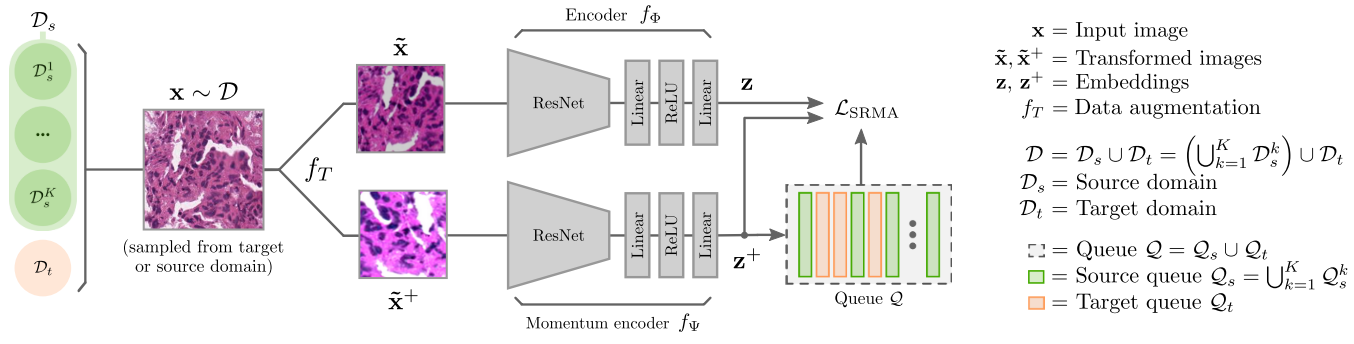Two other predictions generate target pseudo labels for each segmentation network generated by during pseudo labelling.

Implicit pseudo supervision includes semantic feature alignment (SFA) and adaptation ability estimation (AAE). AAE exploits the adaptation ability for each pixel and each network and rectifies the pseudo labels to get $\mathcal{L}_{\text{aae}}$.

Semantic feature alignment (SFA) minimizes the distance of feature centroids between the same class for background categories to get $\mathcal{L}_{\text{back}}$ and maximizes the distance between different categories for foreground types to get $\mathcal{L}_{\text{fore}}$.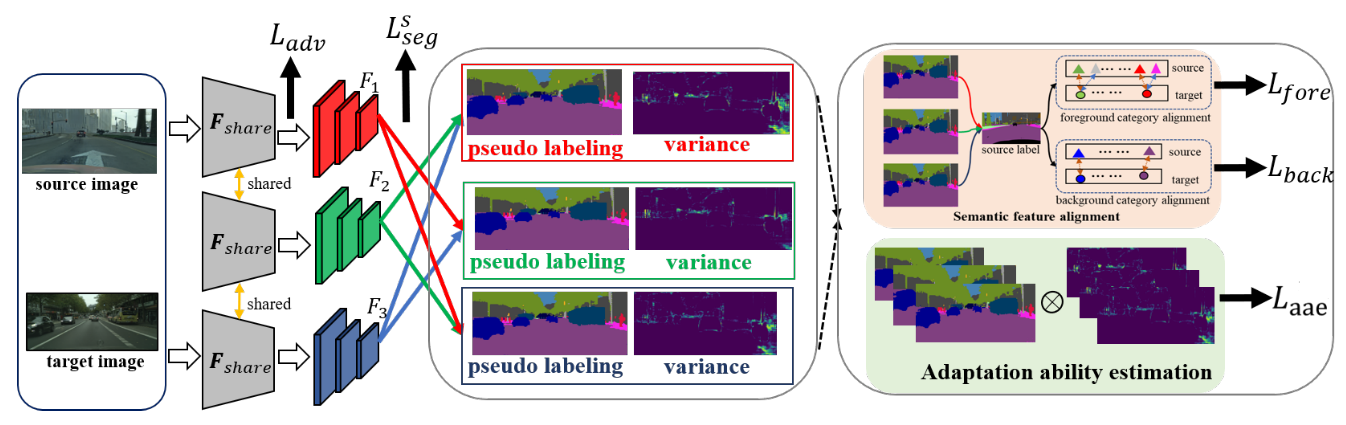## Learn segmentation via clustering

SegDiscover has three steps:

• Creating concept primitives. The images in the dataset are segmented into “concept primitives” (patches/super pixels)
• Concept discovery via primitive clustering. The primitive patches are fed into a pretrained self-supervised network, and potential concepts are discovered by clustering in its latent space.
• Concept refinement via neural network smoothing, the cluster labels are mapped back to the original images to form pseudo segmentation labels. A segmentation network is trained to predict the pseudo labels given the authentic images. The segmentation network refines the learned concepts.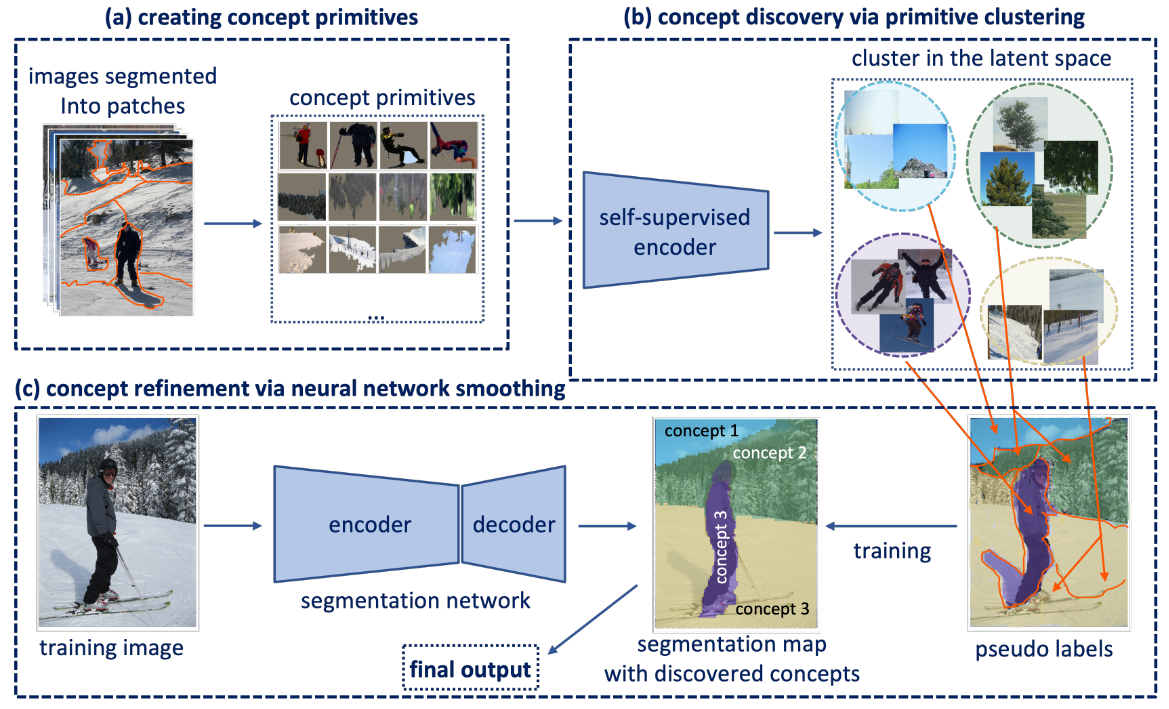The pooled tensor is reshaped and fed into the feature encoder to obtain latent representations. Features (blue points) are clustered in the latent space to get cluster centres through GMM (feature prototypes, shown as red points), and clustering loss can be calculated.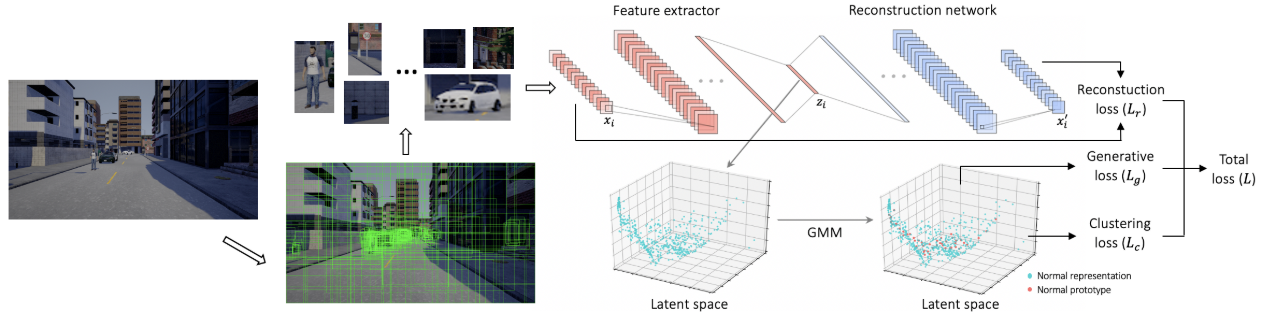## Cluster assignment

In contrastive learning methods applied to instance classification, the features from different transformations of the same images are compared directly. SwAV (swapping assignments between multiple views of the same image) obtain “code” by assigning features to prototype vectors. And then, a swapped prediction trick is applied where the codes received from one data augmented view are predicted using the other view.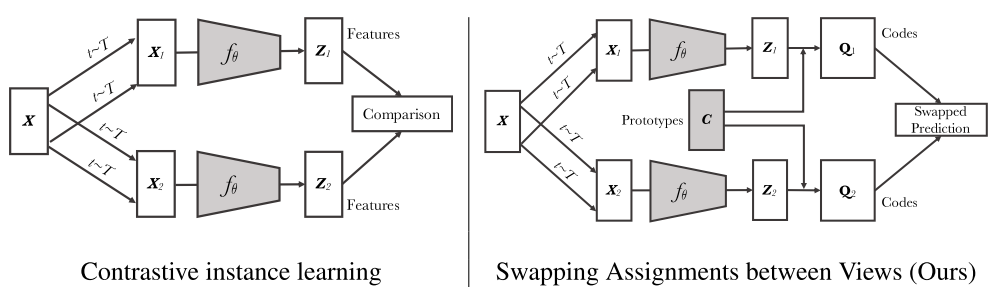# Reference

 Hao Dong, Learning Methods.

 Abbet, C., Studer, L., Fischer, A., Dawson, H., Zlobec, I., Bozorgtabar, B. and Thiran, J.P., 2022. Self-Rule to Multi-Adapt: Generalized Multi-source Feature Learning Using Unsupervised Domain Adaptation for Colorectal Cancer Tissue Detection. Medical Image Analysis, p.102473.

 Xu, W., Wang, Z. and Bian, W., 2022. Unsupervised Domain Adaptation with Implicit Pseudo Supervision for Semantic Segmentation. arXiv preprint arXiv:2204.06747.

 Huang, H., Chen, Z. and Rudin, C., 2022. SegDiscover: Visual Concept Discovery via Unsupervised Semantic Segmentation. arXiv preprint arXiv:2204.10926.

 Liu, J., Qi, X., Su, S., Prescott, T. and Sun, L., 2021, March. Zero-shot Anomalous Object Detection using Unsupervised Metric Learning. In 2021 IEEE/RSJ International Conference on Intelligent Robots and Systems (IROS 2021) Proceedings. Sheffield.

 Dike, H.U., Zhou, Y., Deveerasetty, K.K. and Wu, Q., 2018, October. Unsupervised learning based on artificial neural network: A review. In 2018 IEEE International Conference on Cyborg and Bionic Systems (CBS) (pp. 322-327). IEEE.

 Caron, M., Misra, I., Mairal, J., Goyal, P., Bojanowski, P. and Joulin, A., 2020. Unsupervised learning of visual features by contrasting cluster assignments. Advances in Neural Information Processing Systems, 33, pp.9912-9924.